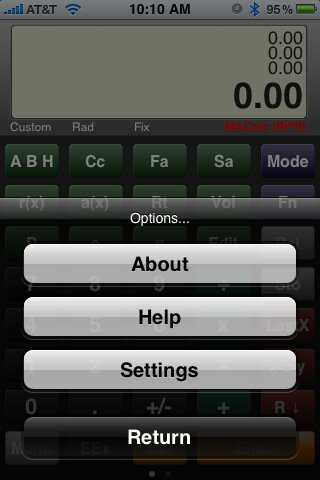Step 1: Install MACalc Pro — Once the application is installed click on the menu button on the bottom left of the screen, then click on settings. (Michael South)

# iPhone Dome Calculator

When the iPhone came out, we could immediately recognize the benefits. We knew that if we were going to make the switch to the iPhone, we would have to come up with a dome calculator.

We found numerous applications on the web with programmable features, but settled on MACalc Pro from TTrix. It is a great calculator with lots of features. Then it was up to David South Jr., my brother, to program it to calculate domes.

Dave has written a great set of dome functions for MACalc Pro. It includes:

• Spherical Domes
• Cc = The circumference
• Fa = The floor area
• Sa = The surface area of the dome
• r(x) = the radius at (x) units above the floor
• a(x) = the area at (x) units above the floor
• Rc = The radius of curvature
• Vol = The volume of the dome
• Sd = The surface distance, or the distance from the top of the dome down to the perimeter.
• Oblate Ellipsoids
• Cc = The circumference
• Fa = The floor area
• Sa = The surface area of the dome
• r(x) = the radius at (x) units above the floor
• a(x) = the area at (x) units above the floor
• Rt = The radius of curvature at the top of the dome.
• Vol = The volume of the dome
• Sd = The surface distance, or the distance from the top of the dome down to the perimeter.
• D = The diameter of the dome
• e = Elliptisity. Its the ratio of the semi major divided by the semi minor axis of an ellipse. We use a maximum elliptisity factor of 1.45. We’ve also found that 1.45 is not only the maximum, but the optimal ratio for oblate ellipses.
• Prolate Ellipsoids
• p = The iterative calculated perimeter of the footing at the floor level
• Fa = The absolute calculation of the floor’s elliptical area
• Sa = The iterative calculation of the surface area of the dome
• ab(x) = Pushes onto the stack the “a” and “b” of the ellipse that intersects the prolate ellipsoid at (x) units above the floor.
• p(x) = The perimeter of the ellipse that intersects the prolate ellipsoid at (x) units above the floor
• a(x) = The elliptical area at (x) units above the floor
• e = Ellipticity which is less than 1.0 for prolate ellipsoids
• a b = Pushes onto the stack the semi-major and semi-minor dimensions of the floor

Installation

To install, watch the video or click on the first picture in the article and the instructions are in the caption.